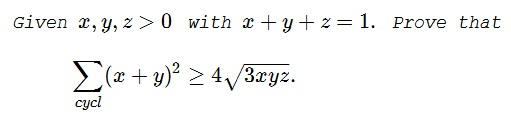# An Inequality with Constraint XX

### Statement### Solution 1

With the Rearrangement inequality,

\displaystyle\begin{align} \sum_{cycl}(x+y)^2 &= 2\sum_{cycl}x^2+2\sum_{cycl}xy\\ &\ge 4\sum_{cycl}xy\ge 4\sqrt{3xyz(x+y+1)}=4\sqrt{3xyz}. \end{align}

### Solution 2

Let $a=y+z,\,$ $b=z+x,\,$ $c=x+y.\,$ Then $a,b,c\,$ are the side lengths of a triangle, with the semiperimeter $s=x+y+z=1,\,$ the area $S=\sqrt{(x+y+z)xyz}=\sqrt{xyz}.\,$ Thus, by the Ionescu-Weitzenbök inequality,

$\displaystyle\sum_{cycl}(x+y)^2=\sum_{cycl}a^2\ge 4\sqrt{3}S=4\sqrt{3xyz}.$

### Solution 3

By the AM-QM inequality

\displaystyle\begin{align} \sum_{cycl}(x+y)^2 &\ge \frac{\displaystyle\left(\sum_{cycl}(x+y)\right)^2}{3}\\ &=\frac{4(x+y+z)^2}{3}, \end{align}

so that

\displaystyle\begin{align} \left(\sum_{cycl}(x+y)^2\right)^2 &\ge \frac{16}{9}\left(x+y+z\right)^4= \frac{16}{9}(x+y+z)^3\\ &\ge\frac{16}{9}\cdot (3\sqrt{xyz})=16\cdot 3\cdot xyz, \end{align}

implying $\displaystyle\sum_{cycl}(x+y)^2\ge 4\sqrt{3xyz}.$

### Solution 4

By the AM-QM inequality

\displaystyle\begin{align} \sum_{cycl}(x+y)^2 &\ge \frac{\displaystyle\left(\sum_{cycl}(x+y)\right)^2}{3}\\ &=\frac{4}{3}(x+y+z)^2=\frac{4}{3}(x+y+z)^{\frac{3}{2}}\\ &=4\sqrt{3}\sqrt{\frac{x+y+z}{3}}\ge 4\sqrt{3}\sqrt{xyz}. \end{align}

### Acknowledgment

The problem, with several solutions, was kindly posted at the CutTheKnotMath facebook page by Dan Sitaru. Dan has previously published the problem at the Romanian Mathematical Magazine. Solution 1 is by Boris Colakovic; Solution 2 is by Dan Sitaru; Solution 3 is by Tri Nitrotoluen; Solution 4 is by Abdelhak Maoukouf.

### Inequalities with the Sum of Variables as a Constraint